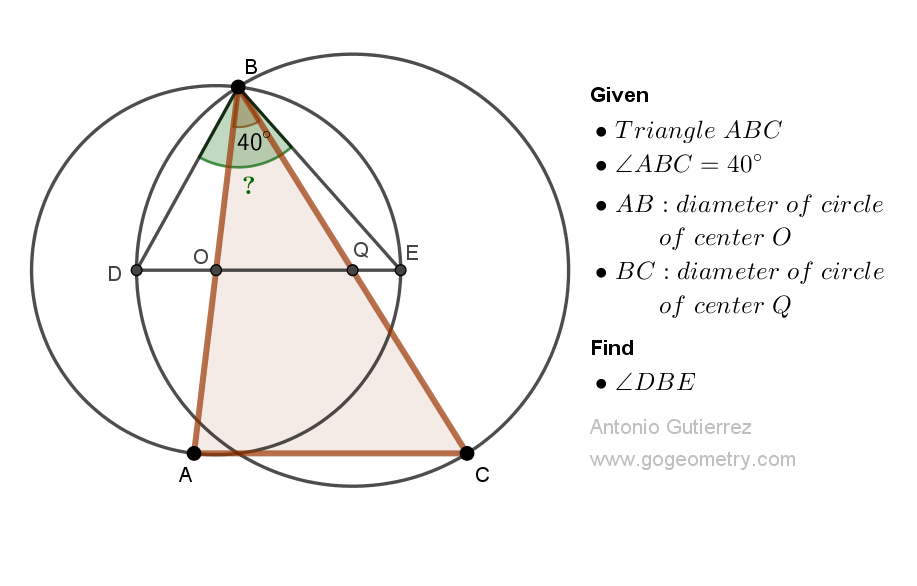# Geometry Problem 1521: Unlock the Secret to Finding the Measure of an Angle in a Triangle with Two Sides as Diameters of Circles. Difficulty Level: High School.

In triangle ABC, angle ABC measures 40 degrees. The circles with diameters AB and BC intersect the line that joins their centers at points D and E as shown in the figure. Find the measure of angle DBE.## Definitions and Suggestions

Concept Definition Theorem
Triangle A three-sided polygon The sum of the three interior angles of any triangle is always equal to 180 degrees
Circle A closed curve consisting of all points that are equidistant from a given point called the center All radii of a circle are congruent (i.e., they have the same length).
Diameter A line segment that passes through the center of a circle and has both endpoints on the circle Diameter theorem - in any circle, the diameter is the longest chord.
Inscribed angle An angle whose vertex is on the circle and whose sides are chords of the circle Inscribed angle theorem - the measure of an inscribed angle in a circle is half the measure of the intercepted arc.
Central angle An angle whose vertex is at the center of a circle and whose sides are radii of the circle Central angle theorem - the measure of a central angle is equal to the measure of the arc it intercepts.

### Thematic Poem: Unlock the Secret to Finding the Measure of an Angle in a Triangle with Circles

In math class, we sit and ponder,
The angles in a triangle, a math wonder,
Two sides as diameters, what could it mean?
Unlock the secret, and the answer will gleam.

With careful study and patient mind,
The measure of the angle, we'll finally find,
We'll learn the methods, the rules, and the tricks,
And soon we'll solve problems with math kicks.

Triangles and circles, a world of math,
A universe of numbers, a complex path,
But fear not, for with each step we take,
We'll unlock the secrets, and never break.

We'll use our minds, and our pencils too,
Draw diagrams and equations anew,
We'll calculate and measure, with precision and care,
And soon the answer will be clear as air.

o let's dive in, and unlock the mystery,
With each step forward, we'll make history,
We'll find the measure, and solve the puzzle,
And in the end, our math skills will dazzle.

Unlock the secret, and learn with glee,
The measure of an angle, for all to see,
We'll conquer math, with a powerful might,
And unlock the secrets, with all our might.

If you're interested in finding more poems with a focus on geometry, you may enjoy this collection: More geometry thematic poems.